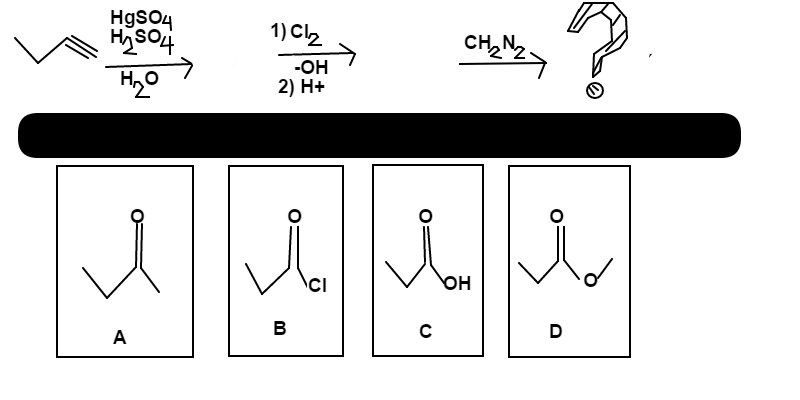# Butyne Transformation

Chemistry Level 2Butyne is mixed in with a solution of $\ce{H_2SO_4}$, $\ce{HgSO_4}$, and $\ce{H2 O}$. This is quickly followed by an addition of $\ce{Cl_2}$ and $\ce{OH^-}$, with a post acid workup. Lastly, diazomethane is added.  Determine which choice; $A$, $B$, $C$, or $D$, is the major product of the above series of reactions.

David's Organic Chemistry Set

David's Physical Chemistry Set

×